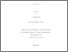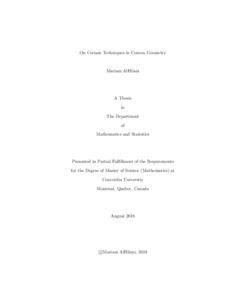Title:

# On Certain Techniques in Convex Geometry

AlHilani, Mariam (2018) On Certain Techniques in Convex Geometry. Masters thesis, Concordia University.Preview
Text (application/pdf)
AlHilani_MSc_F2018.pdf - Accepted Version
3MB

## Abstract

We recall a proof of Mahler's conjecture in R2 and the technique employed to prove it. This technique shows that, by adding new vertices to a convex polygon K, one increases the value of Mahler's functional K --> V (K).V ( K*), thus the minimum of the functional is reached for the convex polygon with least number of vertices. We then study similar techniques in connection to Petty's conjecture in R2 and R3, respectively. Petty's conjecture states that the functional K --> V (Pi K) V^ {n-1}(K) , where K is a compact convex set in Rn, reaches the maximum for the polytope of least vertices in Rn. In R2, we prove that the inequality holds for any convex body K by a similar technique with that of Mahler's problem, which is di�erent from the original proof of Petty's inequality in R2. In R3, we validate the conjecture for a few speci�c cases. More precisely, we compare the value of Petty's functional of a convex body K in Rn, for n = 2 and n = 3, with that of another convex body K' that is obtained by cutting of a vertex of K with a plane, thus introducing more vertices. However, we provide an example that shows that this technique cannot be applied to arbitrary polytopes in R3 to prove Petty's conjecture in this class and then, by approximation, in general.

Divisions: Concordia University > Faculty of Arts and Science > Mathematics and Statistics Thesis (Masters) AlHilani, Mariam Concordia University M. Sc. Mathematics August 2018 Stancu, Alina 984605 Mariam AlHilani 12 Nov 2018 18:00 12 Nov 2018 18:00
All items in Spectrum are protected by copyright, with all rights reserved. The use of items is governed by Spectrum's terms of access.

Repository Staff Only: item control pageResearch related to the current document (at the CORE website)
Back to top